## NCERT Solutions for Class 10 Maths Chapter 5 Arithmetic Progressions Ex 5.2

In this article, we have provided step by step Chapter 5 Ex 5.2 Class 10 Maths NCERT Solutions.

NCERT Solutions for Class 10 Maths Chapter 5 Arithmetic Progressions Ex 5.2 are part of
. Here are we have given Chapter 5 Arithmetic Progressions Class 10 NCERT Solutions Ex 5.2.

 Board CBSE Textbook NCERT Class Class 10 Subject Maths Chapter Chapter 5 Chapter Name Arithmetic Progressions Exercise Ex 5.2 Number of Questions Solved 20 Category NCERT Solutions

## NCERT Solutions for Class 10 Maths Chapter 5 Arithmetic Progressions Ex 5.2

You can also Read Latest NCERT Solutions for Class 10 Maths Chapter-wise to help you to revise the complete Syllabus and score more marks in your examinations.

Page No: 105

Question 1. Fill in the blanks in the following table, given that a is the first term, d the common difference and an the nth term of the A.P.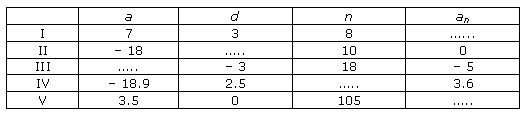Solution :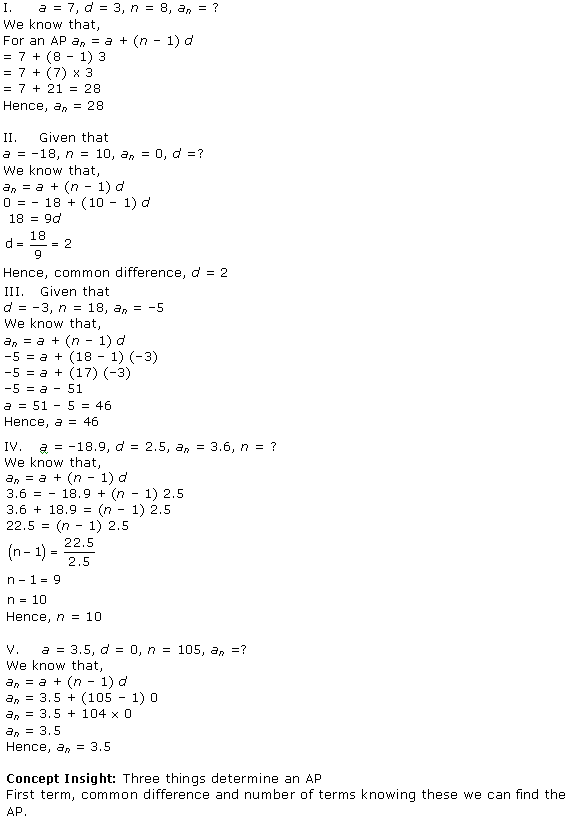Question 2. Choose the correct choice in the following and justify
(i) 30th term of the A.P: 10, 7, 4, …, is
(A) 97 (B) 77 (C) −77 (D.) −87

(ii) 11th term of the A.P. -3, -1/2, ,2 …. is
(A) 28 (B) 22 (C) – 38 (D) -48×1/2

Solution :Question 3. In the following APs find the missing term in the boxes.Solution :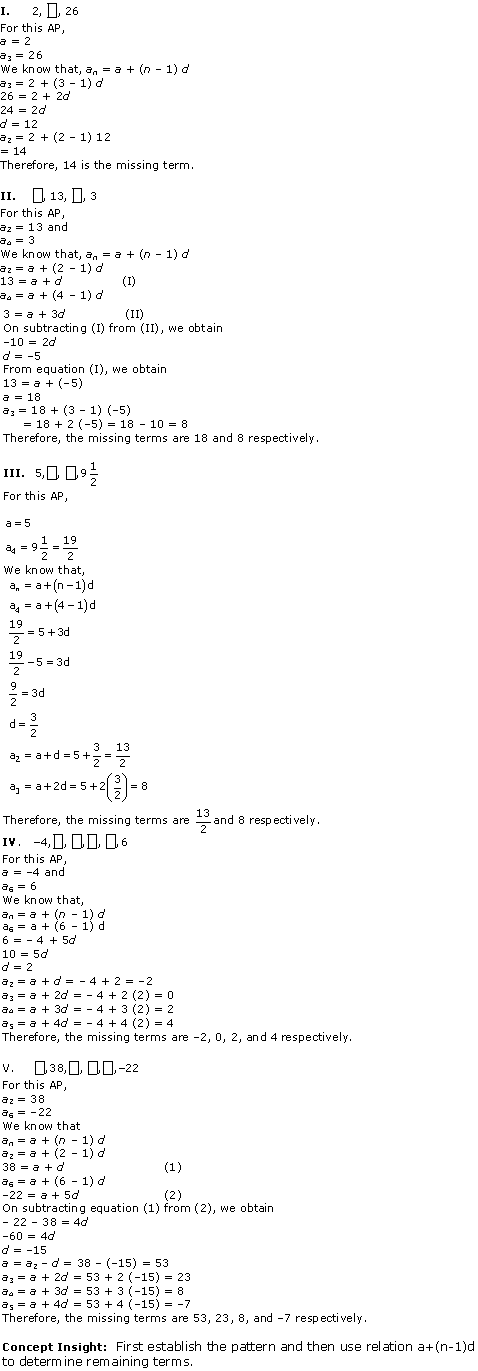Question 4. Which term of the A.P. 3, 8, 13, 18, … is 78?

Solution :Question 5. Find the number of terms in each of the following A.P.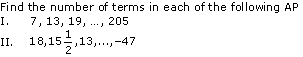Solution :Question 6. Check whether -150 is a term of the A.P. 11, 8, 5, 2, …

Solution :Question 7. Find the 31st term of an A.P. whose 11th term is 38 and the 16thterm is 73.

Solution :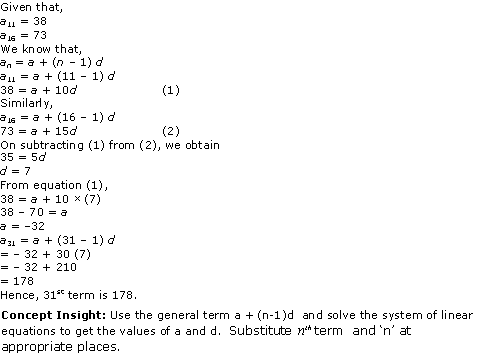Question 8. An A.P. consists of 50 terms of which 3rd term is 12 and the last term is 106. Find the 29th term.

Solution :Question 9. If the 3rd and the 9th terms of an A.P. are 4 and − 8 respectively. Which term of this A.P. is zero.

Solution :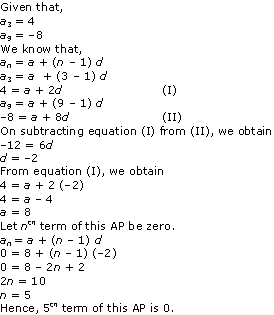Question 10. If 17th term of an A.P. exceeds its 10th term by 7. Find the common difference.

Solution :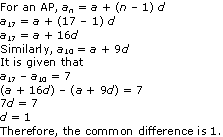Question 11. Which term of the A.P. 3, 15, 27, 39, … will be 132 more than its 54th term?

Solution :Question 12. Two APs have the same common difference. The difference between their 100th term is 100, what is the difference between their 1000th terms?

Solution :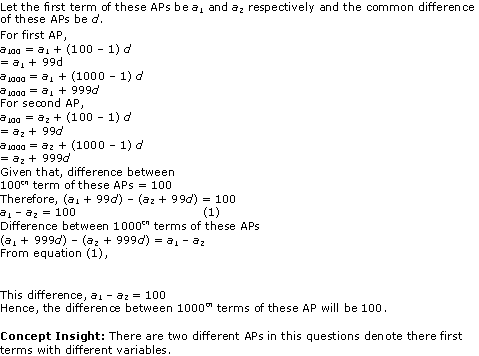Question 13. How many three digit numbers are divisible by 7?

Solution :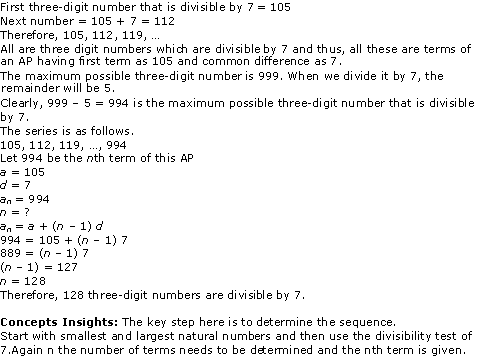Question 14. How many multiples of 4 lie between 10 and 250?

Solution :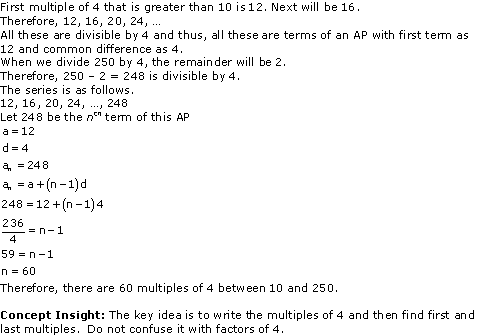Question 15. For what value of n, are the nth terms of two APs 63, 65, 67, and 3, 10, 17, … equal?

Solution :Question 16. Determine the A.P. whose third term is 16 and the 7th term exceeds the 5th term by 12.

Solution :Page No: 107

Question 17. Find the 20th term from the last term of the A.P. 3, 8, 13, …, 253.

Solution :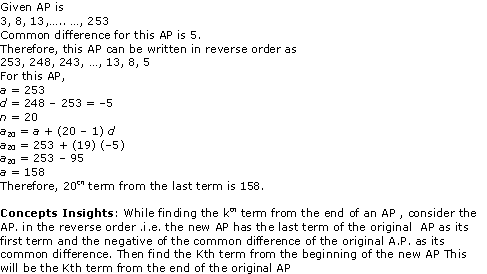Question 18. The sum of 4th and 8th terms of an A.P. is 24 and the sum of the 6th and 10th terms is 44. Find the first three terms of the A.P.

Solution :Question 19. Subba Rao started work in 1995 at an annual salary of Rs 5000 and received an increment of Rs 200 each year. In which year did his income reach Rs 7000?

Solution :Question 20. Ramkali saved Rs 5 in the first week of a year and then increased her weekly saving by Rs 1.75. If in the nth week, her week, her weekly savings become Rs 20.75, find n.

Solution :We hope the NCERT Solutions for Class 10 Maths Chapter 5 Arithmetic Progressions Ex 5.2 help you. If you have any query regarding NCERT Solutions for Class 10 Maths Chapter 5 Arithmetic Progressions Ex 5.2, drop a comment below and we will get back to you at the earliest.

## NCERT Solutions for Class 10 Maths Chapter 14 Statistics Ex 14.4

Get the comprehensive Chapter 14 Ex 14.4 Class 10 Maths NCERT Solutions from here and score good marks in CBSE Class 10 Board Examinations.

NCERT Solutions for Class 10 Maths Chapter 14 Statistics Ex 14.4 are part of NCERT Solutions for Class 10 Maths. Here are we have given Chapter 14 Statistics Class 10 NCERT Solutions Ex 14.4.

 Board CBSE Textbook NCERT Class Class 10 Subject Maths Chapter Chapter 14 Chapter Name Statistics Exercise Ex 14.4 Number of Questions Solved 3 Category NCERT Solutions

## NCERT Solutions for Class 10 Maths Chapter 14 Statistics Ex 14.4

Question 1.
The following distribution gives the daily income of 50 workers of a factory.

 Daily income (in Rs) 100 – 120 120 – 140 140 – 160 160 – 180 180 – 200 Number of workers 12 14 8 6 10

Convert the distribution above to a less than type cumulative frequency distribution, and draw its ogive.

Solution:

We can find frequency distribution table of less than type as following –

 Daily income  (in Rs)(upper class limits) Cumulative frequency Less than 120 12 Less than 140 12 + 14 = 26 Less than 160 26 + 8 = 34 Less than 180 34 + 6 = 40 Less than 200 40 + 10 = 50

Now taking upper class limits of class intervals on x-axis and their respective frequencies on y-axis we can draw its ogive as following –Question 2.
During the medical check-up of 35 students of a class, their weights were recorded as follows:

 Weight (in kg) Number of students Less than 38 0 Less than 40 3 Less than 42 5 Less than 44 9 Less than 46 14 Less than 48 28 Less than 50 32 Less than 52 35

Draw a less than type ogive for the given data. Hence obtain the median weight from the graph verify the result by using the formula.

Solution:
The given cumulative frequency distributions of less than type is –

 Weight  (in kg)  upper class limits Number of students  (cumulative frequency) Less than 38 0 Less than 40 3 Less than 42 5 Less than 44 9 Less than 46 14 Less than 48 28 Less than 50 32 Less than 52 35

Now taking upper class limits on x-axis and their respective cumulative frequency on y-axis we may draw its ogive as following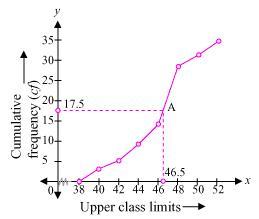Now mark the point A whose ordinate is 17.5 its x-coordinate is 46.5. So median of this data is 46.5.
We may observe that difference between two consecutive upper class limits is 2. Now we may obtain class marks with their respective frequencies as below

 Weight  (in kg) Frequency (f) Cumulative frequency Less than 38 0 0 38 – 40 3 –  0 = 3 3 40 – 42 5 – 3 = 2 5 42 – 44 9 – 5 = 4 9 44 – 46 14 – 9 = 5 14 46 – 48 28 – 14 = 14 28 48 – 50 32 – 28 = 4 32 50 – 52 35 – 32 = 3 35 Total (n) 35

Now the cumulative frequency just greater thanis 28 belonging to class interval 46 –  48
Median class = 46 – 48
Lower class limit (l) of median class = 46
Frequency (f) of median class = 14
Cumulative frequency (cf) of class preceding median class = 14
Class size (h) = 2So median of this data is 46.5
Hence, value of median is verified.

Question 3.
The following table gives production yield per hectare of wheat of 100 farms of a village.

 Production yield (in kg/ha) 50 – 55 55 – 60 60 – 65 65 – 70 70 – 75 75 – 80 Number of farms 2 8 12 24 38 16

Change the distribution to a more than type distribution and draw ogive.

Solution:
We can obtain cumulative frequency distribution of more than type as following –
 Production yield  (lower class limits) Cumulative frequency more than or equal to 50 100 more than or equal to 55 100 – 2 = 98 more than or equal to 60 98 – 8 = 90 more than or equal to 65 90 – 12 = 78 more than or equal to 70 78 – 24 = 54 more than or equal to 75 54 – 38 = 16

Now taking lower class limits on x-axis and their respective cumulative frequencies on y-axis we can obtain its ogive as following.We hope the NCERT Solutions for Class 10 Maths Chapter 14 Statistics Ex 14.4 help you. If you have any query regarding NCERT Solutions for Class 10 Maths Chapter 14 Statistics Ex 14.4, drop a comment below and we will get back to you at the earliest.

## NCERT Solutions for Class 10 Maths Chapter 1 Real Numbers Ex 1.4

NCERT Maths Solutions for Ex 1.4 Class 10 Real Numbers is the perfect guide to boost up your preparation during CBSE 10th Class Maths Examination.

NCERT Solutions for Class 10 Maths Chapter 1 Real Numbers Ex 1.4 are part of . Here are we have given Chapter 1 Real Numbers Class 10 NCERT Solutions Ex 1.4.

 Board CBSE Textbook NCERT Class Class 10 Subject Maths Chapter Chapter 1 Chapter Name Real Numbers Exercise Ex 1.4 Number of Questions Solved 3 Category NCERT Solutions

## NCERT Solutions for Class 10 Maths Chapter 1 Real Numbers Ex 1.4

Page No: 17

Question 1
Without actually performing the long division, state whether the following rational numbers will have a terminating decimal expansion or a non-terminating repeating decimal expansion:
(i) 13/3125
(ii) 17/8
(iii) 64/455
(iv) 15/1600
(v) 29/343
(vi) 23/23 × 52
(vii) 129/22 × 57 × 75
(viii) 6/15
(ix) 35/50
(x) 77/210

Solution: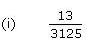3125 = 55
The denominator is of the form 5m.8 = 23
The denominator is of the form 2m.455 = 5 x 7 x 13
Since the denominator is not in the form 2m x 5n, and it also contains 7 and 13 as its factors, its decimal expansion will be non-terminating repeating.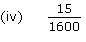1600 = 26 × 52
The denominator is of the form 2m x 5n.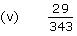343 = 73
Since the denominator is not in the form 2m x 5n, and it has 7 as its factor,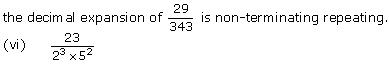The denominator is of the form 2m x 5n. Hence, the decimal expansion of is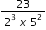terminating.

(vii)Since the denominator is not of the form 2m  5n, and it also has 7 as itsThe denominator is of the form 5n.
10 = 2 x 5
The denominator is of the form 2m x 5n.30 = 2 x 3 x 5
Since the denominator is not of the form 2m × 5n, and it also has 3 as its factors,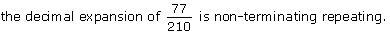Concept Insight: The concept used in this problem is that The decimal expansion of rational number p/q where p and q are coprime numbers, terminates if and only if the prime factorization of q is of the form 2n5m, where n and m are non negative integers. Do not forget that 0 is also a non negative integer so n or m can take value 0.
Generally, mistake is committed in identifying terminating decimals when either of the two prime numbers  2 or 5 is appearing in the prime factorization.

Page No: 18

Question 2
Write down the decimal expansions of those rational numbers in Question 1 above which have terminating decimal expansions.

Solution: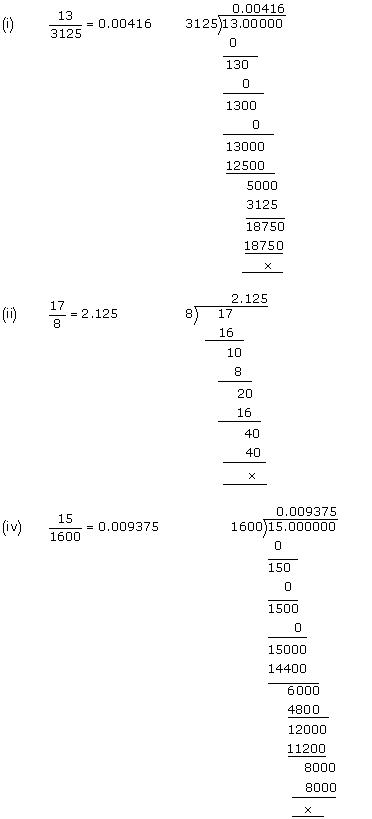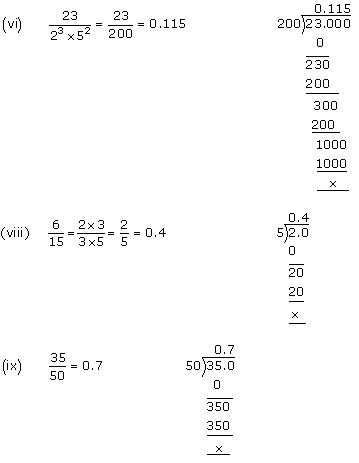Concept Insight:
This is based on performing the long division and expressing the rational number in the decimal form learned in lower classes.

Question 3
The following real numbers have decimal expansions as given below. In each case, decide whether they are rational or not. If they are rational, and of the form p, q you say about the prime factors of q?
(i) 43.123456789
(ii) 0.120120012000120000…
(iii) 43.123456789

Solution:
(i) 43.123456789
Since this number has a terminating decimal expansion, it is a rational number of the form  p\q and q is of the form 2m x 5n,
i.e., the prime factors of q will be either 2 or 5 or both.

(ii) 0.120120012000120000…
The decimal expansion is neither terminating nor recurring. Therefore, the given number is an irrational number.

(iii) 43.123456789
Since the decimal expansion is non-terminating recurring, the given number is a rational number of the form p/q and q is not of the form 2m x 5n  i.e., the prime factors of q will also have a factor other than 2 or 5.

Concept Insight: The concept used in this problem is that, If the decimal expansion of rational number p\q, [where p and q are coprime numbers] terminates, then prime factorization of q is of the form 2n5m, where n and m are non negative integers.

We hope the NCERT Solutions for Class 10 Maths Chapter 1 Real Numbers Ex 1.4 help you. If you have any query regarding NCERT Solutions for Class 10 Maths Chapter 1 Real Numbers Ex 1.4, drop a comment below and we will get back to you at the earliest.

## NCERT Solutions for Class 10 Maths Chapter 8 Introduction to Trigonometry Ex 8.2

Get the comprehensive Chapter 8 Ex 8.2 Class 10 Maths NCERT Solutions from here and score good marks in CBSE Class 10 Board Examinations

NCERT Solutions for Class 10 Maths Chapter 8 Introduction to Trigonometry Ex 8.2 are part of NCERT Solutions for Class 10 Maths. Here are we have given Chapter 8 Introduction to Trigonometry Class 10 NCERT Solutions Ex 8.2.

 Board CBSE Textbook NCERT Class Class 10 Subject Maths Chapter Chapter 8 Chapter Name Introduction to Trigonometry Exercise Ex 8.2 Number of Questions Solved 4 Category NCERT Solutions

## NCERT Solutions for Class 10 Maths Chapter 8 Introduction to Trigonometry Ex 8.2

Page No: 187

Question 1. Evaluate the following :
(i) sin 60° cos 30° + sin 30° cos 60°
(ii) 2 tan245° + cos230° – sin260°
(iii) $$\frac { cos45^{ 0 } }{ sec{ 30 }^{ 0 }+cosec{ 30 }^{ 0 } }$$
(iv) $$\frac { sin{ 30 }^{ 0 }+tan45^{ 0 }-cosec{ 60 }^{ 0 } }{ sec{ 30 }^{ 0 }+cosec{ 60^{ 0 } }+cot{ 45 }^{ 0 } }$$
(v) $$\frac { 5cos^{ 2 }{ 60 }^{ 0 }+4sec^{ 2 }{ 30 }^{ 0 }-tan^{ 2 }{ 45 }^{ 0 } }{ sin^{ 2 }{ 30 }^{ 0 }+cos^{ 2 }{ 30 }^{ 0 } }$$

Solution: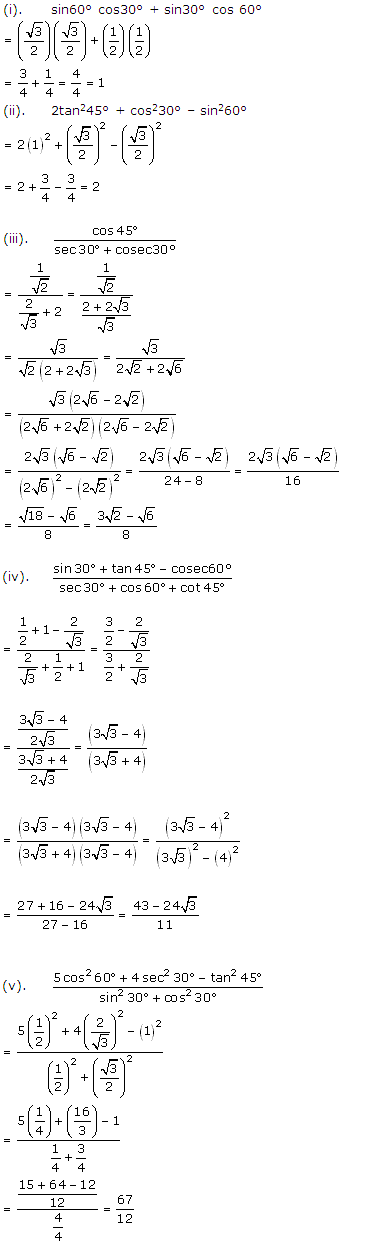Question 2. Choose the correct option and justify your choice :
(i) $$\frac { 2tan{ 30 }^{ 0 } }{ 1+tan^{ 2 }{ 30 }^{ 0 } } =$$
(A) sin 60° (B) cos 60° (C) tan 60° (D) sin 30°

(ii) $$\frac { 1-tan{ ^{ 2 }45 }^{ 0 } }{ 1+tan{ ^{ 2 }45 }^{ 0 } } =$$
(A) tan 90° (B) 1 (C) sin 45° (D) 0

(iii) sin 2A = 2 sin A is true when A =
(A) 0° (B) 30° (C) 45° (D) 60°

(iv) $$\frac { 2tan{ 30 }^{ 0 } }{ 1-tan{ ^{ 2 }30 }^{ 0 } } =$$
(A) cos 60° (B) sin 60° (C) tan 60° (D) sin 30°

Solution:Question 3. If tan (A + B) = √3 and tan (A – B) = 1/√3; 0° < A + B ≤ 90°; A > B, find A and B.

Solution: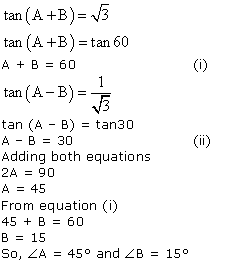Question 4. State whether the following are true or false. Justify your answer.
(i) sin (A + B) = sin A + sin B.
(ii) The value of sin θ increases as θ increases.
(iii) The value of cos θ increases as θ increases.
(iv) sin θ = cos θ for all values of θ.
(v) cot A is not defined for A = 0°.

Solution:We hope the NCERT Solutions for Class 10 Maths Chapter 8 Introduction to Trigonometry Ex 8.2 help you. If you have any query regarding NCERT Solutions for Class 10 Maths Chapter 8 Introduction to Trigonometry Ex 8.2, drop a comment below and we will get back to you at the earliest.

## NCERT Solutions for Class 10 Maths Chapter 2 Polynomials Ex 2.3

NCERT Maths Solutions for Ex 2.3 class 10 Polynomials is the perfect guide to boost up your preparation during CBSE 10th Class Maths Examination.

NCERT Solutions for Class 10 Maths Chapter 2 Polynomials Ex 2.3 are part of . Here are we have given Chapter 2 Polynomials Class 10 NCERT Solutions Ex 2.3.

 Board CBSE Textbook NCERT Class Class 10 Subject Maths Chapter Chapter 2 Chapter Name Polynomials Exercise Ex 2.3 Number of Questions Solved 5 Category NCERT Solutions

## NCERT Solutions for Class 10 Maths Chapter 2 Polynomials Ex 2.3

Page No: 36

Question 1. Divide the polynomial p(x) by the polynomial g(x) and find the quotient and remainder in each of the following: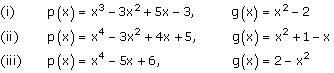Solution:Quotient = x – 3
Remainder = 7x – 9Quotient = x2 + x – 3
Remainder = 8Quotient = -x2 – 2
Remainder = -5x + 10

Concept Insight: While dividing one polynomial by another, first arrange the polynomial in descending powers of the variable. In the process of division, be careful about the signs of the coefficients of the terms of the polynomials. After performing division, one can check his/her answer obtained by the division algorithm which is as below:
Dividend = Divisor x Quotient + Remainder
Also, remember that the quotient obtained is a polynomial only.

Question 2. Check whether the first polynomial is a factor of the second polynomial by dividing the
second polynomial by the first polynomial:Solution:
The polynomial 2t4 + 3t3 – 2t2 – 9t – 12  can be divided by the polynomial t2 – 3 = t2 + 0.t – 3 as follows:Since the remainder is 0, t² – 3 is a factor of 2t4 + 3t3 – 2t2 – 9t – 12 .
(ii) The polynomial 3x4 + 5x3 – 7x2 + 2x + 2 can be divided by the polynomial x2 + 3x + 1 as follows:
Since the remainder is 0, x² + 3x + 1 is a factor of 3x4 + 5x3 – 7x2 + 2x + 2
(iii) The polynomial x5 – 4x3 + x2 + 3x + 1 can be divided by the  polynomial x3 – 3x + 1 as follows:Since the remainder is not equal to 0, x3 – 3x + 1 is not a factor of x5 – 4x3 + x2 + 3x + 1.

Concept Insight: A polynomial g(x) is a factor of another polynomial p(x) if the remainder obtained on dividing p(x) by g(x) is zero and not just a constant. While changing the sign, make sure you do not change the sign of the terms which were not involved in the previous operation. For example in the first step of (iii), do not change the sign of 3x + 1.

Question 3. Obtain all other zeroes of 3x4 + 6x3 – 2x2 – 10x – 5, if two of its zeroes are √(5/3) and – √(5/3).

Solution:
Let p(x) = 3x4 + 6x3 – 2x2 – 10x -5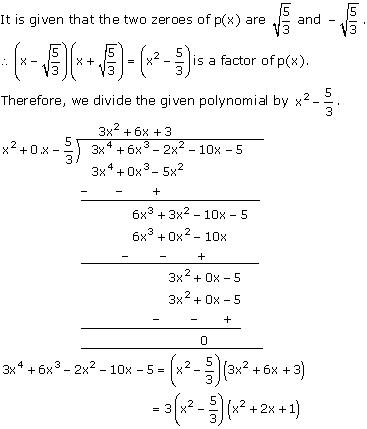Now, x² + 2x + 1 = (x + 1)2
So, the two zeroes of  x² + 2x + 1 are -1 and -1.Concept Insight:  Remember that if (x – a) and (x – b) are factors of a polynomial, then (x – a)(x – b) will also be a factor of that polynomial. Also, if a is a zero of a polynomial p(x), where degree of p(x) is greater than 1, then (x – a) will be a factor of p(x), that is when p(x) is divided by (x – a), then the remainder obtained will be 0 and the quotient will be a factor of the polynomial p(x). To cross check your answer number of zeroes of the polynomial will be less than or equal to the degree of the polynomial.

Question 4. On dividing x3 – 3x2 + x + 2 by a polynomial g(x), the quotient and remainder were x – 2 and
-2x + 4, respectively. Find g(x).

Solution:
Divided, p(x) = x3 – 3x2 + x + 2
Quotient = (x – 2)
Remainder = (-2x + 4)
Let g(x) be the divisor.
According to the division algorithm,
Dividend = Divisor x Quotient + RemainderConcept Insight: When a polynomial is divided by any other non-zero polynomial, then it satisfies the division algorithm which is as below:
Dividend = Divisor x Quotient + Remainder
Divisor x Quotient = Dividend – Remainder
So, from this relation, the divisor can be obtained by dividing the result of (Dividend – Remainder) by the quotient.

Question 5. Give examples of polynomial p(x), g(x), q(x) and r(x), which satisfy the division algorithm and
(i) deg p(x) = deg q(x)
(ii) deg q(x) = deg r(x)
(iii) deg r(x) = 0

Solution:
According to the division algorithm, if p(x) and g(x) are two polynomials with g(x) ≠ 0, then we can find polynomials q(x) and r(x) such that
p(x) = g(x) x q(x) + r(x), where r(x) = 0 or degree of r(x) < degree of g(x).

(i)  Degree of quotient will be equal to degree of dividend when divisor is constant.
Let us consider the division of 18x2 + 3x + 9  by 3.
Here, p(x) = 18x2 + 3x + 9  and g(x) = 3
q(x) = 6x2 + x + 3  and r(x) = 0
Here, degree of p(x) and q(x) is the same which is 2.
Checking:
p(x) = g(x) x q(x) + r(x)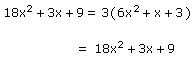Thus, the division algorithm is satisfied.

(ii)  Let us consider the division of 2x4 + 2x by 2x3,
Here, p(x) = 2x4 + 2x and g(x) = 2x3
q(x) = x and r(x) = 2x
Clearly, the degree of q(x) and r(x) is the same which is 1.
Checking,
p(x) = g(x) x q(x) + r(x)
2x4 + 2x =  (2x3 ) x x  + 2x
2x4 + 2x = 2x4 + 2x
Thus, the division algorithm is satisfied.

(iii)    Degree of remainder will be 0 when remainder obtained on division is a constant.
Let us consider the division of 10x3 + 3 by 5x2.
Here, p(x) = 10x3 + 3 and g(x) = 5x2
q(x) = 2x and r(x) = 3
Clearly, the degree of r(x) is 0.
Checking:
p(x) = g(x) x q(x) + r(x)
10x3 + 3 = (5x2 ) x 2x  +  3
10x3 + 3 = 10x3 + 3
Thus, the division algorithm is satisfied.

Concept Insight: In order to answer such type of questions, one should remember the division algorithm. Also, remember the condition on the remainder polynomial r(x). The polynomial r(x) is either 0 or its degree is strictly less than g(x). The answer may not be unique in all the cases because there can be multiple polynomials which satisfy the given conditions.

We hope the NCERT Solutions for Class 10 Maths Chapter 2 Polynomials Ex 2.3 help you. If you have any query regarding NCERT Solutions for Class 10 Maths Chapter 2 Polynomials Ex 2.3, drop a comment below and we will get back to you at the earliest.